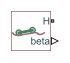Road profile with varying elevation and friction properties

• Library:
• Simscape / Driveline / Tires & Vehicles

•## Description

The Road Profile block represents a road profile with varying elevation and friction properties. As a vehicle travels, the axle parameters and the position of the center of gravity (CG) determine the position of the front and rear axles. The block uses the axle positions to compute vehicle angle and, optionally, tire friction coefficients. The block models friction using either Magic Formula or kinetic and static friction coefficients.

### Variables

To set the priority and initial target values for the block variables prior to simulation, use the Initial Targets section in the block dialog box or Property Inspector. For more information, see Set Priority and Initial Target for Block Variables and Initial Conditions for Blocks with Finite Moist Air Volume.

Nominal values provide a way to specify the expected magnitude of a variable in a model. Using system scaling based on nominal values increases the simulation robustness. Nominal values can come from different sources, one of which is the Nominal Values section in the block dialog box or Property Inspector. For more information, see Modify Nominal Values for a Block Variable.

## Ports

### Conserving

expand all

Conserving port associated with the horizontal motion. Connect to the H port of the Vehicle Body block.

### Output

expand all

Physical signal output port for the friction coefficients of the front axle.

The vector contains four elements for the Magic Formula friction model or two elements for the friction coefficients friction model. Connect to the M port of a front axle Tire (Friction Parameterized) or Tire (Magic Formula) block.

This port is visible only when the Friction output parameter is set to one of these values:

• Physical signal Magic Formula coefficients

• Physical signal friction coefficients

Physical signal output port for the friction coefficients of the rear axle.

The vector contains four elements for the Magic Formula friction model or two elements for the friction coefficients friction model. Connect to the M port of a rear axle Tire (Friction Parameterized) or Tire (Magic Formula) block.

#### Dependencies

This port is visible only when the Friction parameter Friction output is set to one of these values:

• Physical signal Magic Formula coefficients

• Physical signal friction coefficients

Physical signal output port for the vehicle angle.

## Parameters

expand all

### Main

Horizontal distance from the center of gravity (CG) to the front axle. Specify the same value as is specified for the Horizontal distance from CG to front axle parameter of the connected Vehicle Body block.

Horizontal distance from the center of gravity (CG) to the rear axle. Specify the same value as is specified for the Horizontal distance from CG to rear axle parameter of the connected Vehicle Body block.

Table lookup corresponding to the distance that the CG of the vehicle moves relative to the reference frame x-axis. For example the figure shows a horizontal profile of [-10, 0, 10]. Table lookup extrapolation is nearest.Table lookup corresponding to the distance that the CG of the vehicle moves relative to the reference frame y-axis. For example the figure shows a vertical profile of [2, 0, 5]. Table lookup extrapolation is nearest.### Friction

Type of friction coefficient that the block outputs. Select ```Physical signal Magic Formula coefficients``` to vary friction for Tire (Magic Formula) blocks. Select ```Physical signal friction coefficients``` to vary friction for Tire (Friction Parameterized) blocks.

#### Dependencies

Setting this parameter to Physical signal Magic Formula coefficients or Physical signal friction coefficients makes the MF and MR ports and associated Friction parameters visible.

Friction profile horizontal distance.

#### Dependencies

This parameter is visible only when the Friction parameter Friction output is set to one of these values:

• Physical signal Magic Formula coefficients

• Physical signal friction coefficients

Magic Formula coefficients specified as an n-by-4 matrix, where n is the number of points in the vector specified by the Horizontal distance for friction profile parameter.

#### Dependencies

This parameter is visible only when the Friction parameter Friction output is set to Physical signal Magic Formula coefficients.

Magic Formula coefficients specified as an n-by-4 matrix, where n is the number of points in the vector specified by the Horizontal distance for friction profile parameter.

#### Dependencies

This parameter is visible only when the Friction parameter Friction output is set to Physical signal Magic Formula coefficients.

Front axle static and kinetic friction coefficients specified as an n-by-2 matrix, where n is the number of points in the vector specified by the Horizontal distance for friction profile parameter.

#### Dependencies

This parameter is visible only when the Friction parameter Friction output is set to Physical signal friction coefficients.

Rear axle static and kinetic friction coefficients specified as an n-by-2 matrix, where n is the number of points in the vector specified by the Horizontal distance for friction profile parameter.

#### Dependencies

This parameter is visible only when the Friction parameter Friction output is set to Physical signal friction coefficients.

## Version History

Introduced in R2019a